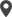Enable Dark Mode!By: Aswani VT

# How to Use Common Python String Methods

## Technical

Python string methods are some set of built-in functions that can be used on strings.
Python strings are enclosed by either single or double quotation marks.
That is, ‘My world’ is the same as “My world.” One can display python strings using the print() function. Multiline strings are assigned using triple quotes.
Python strings are represented using Unicode characters which are actually arrays of bytes. Thus we can loop/iterate through the characters of a string using a  “for”  loop. In Order to access an element of a string, we can use square brackets as below,
Eg:
x = “My World”
print(x)
output: y
The length of the string can be achieved by using the len() function.
E.g.:
x= “My World”
print(len(x))
output: 8
Python string methods return new values, and the original string remains unchanged.
Let’s see some of the common built-in methods in python with examples,
1. capitalize()
The First letter of the string is capitalized.
x = "my world."
y = x.capitalize()
print (y)
output:  My world.
2. casefold()
String is converted to lowercase.
Eg:
x = "MY WORLD."
y = x.capitalize()
print (y)
output:  my world.
3. center()
The function returns a centered string.
Eg:
We can use ‘p as the padding character.
x = "carrot"
y = x.center(20, "P")
print(y)
Output: PPPPPPPcarrotPPPPPPP
4. count()
This function returns the number of occurrences of a specified value.
Eg:
x = "I love bananas, banana are my favorite fruit"
y = x.count("banana")
print(y)
output:
5. encode()
This function returns an encoded string.
Eg:
x = "My name is John"
y = x.encode()
print(y)
output:  b'My name is John'
6. endswith()
This function returns true if the string endswith given value.
Eg:
x = "My name is John."
y = x.endswith(".")
print(y)
output: True
7. expandtabs()
This function sets the tab size of the string.
Eg:
x = "W\to\tr\tl\td"
y =  x.expandtabs(4)
print(y)
output: W   o   r   l   d
8. find()
This function searches the given value in the string and returns the position of the given value.
Eg:
x = "My name is John."
y = x.find("name")
print(y)
output: 3
9. format()
This function formats the given value in the string.
Eg:
x = "I have {num:.2f} rupees!"
print(x.format(num = 20))
output: I have 20.00 rupees!

 10 isalnum() This function checks if all characters are alphanumeric and return true. 11 isalpha() This function checks if all characters are in the alphabet and return true. 12 isascii() This function checks if all characters are ASCII and return true. 13 isdecimal() This function checks if all characters are digits and return true. 14 isidentifier() This function checks if the string is an identifier and returns true. 15 islower() This function checks if all characters are lowercase and return true. 16 isnumeric() This function checks if all characters are numeric and return true. 17 isprintable() This function checks if all characters are printable and return true. 18 isspace() This function checks if all characters are whitespaces and return true. 19 istitle() This function checks if the string follows the rules of a title and returns true. 20 isupper() This function checks if all characters are uppercase and return true. 21 lower() This function converts all characters of the string into lowercase. 22 upper() This function converts all characters of the string into uppercase. 23 title() This function converts the first character of each word in the string into uppercase.
24. index()
This function returns the position of the specified value.
Eg:
x = "My world"
y = x.index("world")
print(y)
output: 3
25. join()
This function joins the elements of an iterable into a single string.
Eg:
x = ("a", "b", "c")
y = "#".join(x)
print(y)
output: a#b#c
26.ljust()
This function returns the left justified version of given text.
Eg:
x = "Life"
y = x.ljust(10)
print(y, "is amazing.")
output: Life       is amazing.
27. lstrip()
This function returns a left-striped version of a given string.
Eg:
x = " life "
y = x.lstrip()
print("My", y ,"is beautiful")
output: My is beautiful
 28 maketrans() Used with the translate method to replace specified characters by forming a mapping. 29 partition() Searches for a given element and parts the string into three. 30 replace() The given value is replaced with the specified character 31 rfind() Returns the last position of the specified value. 32 rindex() Returns the last position of the specified value. 33 rjust() This function returns the right justified version of the given text. 34 rstrip() This function returns a right-striped version of a given string. 35 split() The given string is split at a specified value and returned as a list 36 splitlines() The splitting occurs at line breaks 37 startswith() This function returns True if a string starts with the given value 38 strip() A trimmed version of the string is returned 39 swapcase() Lowercase letters converted into upper and uppercase content in lower 40 translate() The translated string is returned 41 zfill() Fills the string with a given number of zeros at the starting

Above are the most common python string methods with their usage. Learn more about them by trying out each function.

If you need any assistance in odoo, we are online, please chat with us.

### Recent Posts

#### Tree View Decoration Attributes in Odoo 17Calicut

Cybrosys Technologies Pvt. Ltd.
Neospace, Kinfra Techno Park
Kakkancherry, Calicut
Kerala, India - 673635London

Cybrosys Limited
Alpha House,
100 Borough High Street, London,
SE1 1LB, United KingdomKochi

Cybrosys Technologies Pvt. Ltd.
1st Floor, Thapasya Building,
Kochi, India - 682030.Bangalore

Cybrosys Techno Solutions
The Estate, 8th Floor,